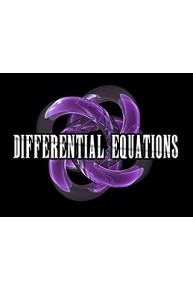# Watch Differential Equations

This course is a study of ordinary differential equations with applications in the physical and social sciences. Topics include: Definitions and Terminology, Solutions, Implicit Solutions, Families of Solutions and Systems of Differential Equations. Differential Equations is a series that is currently running and has 1 seasons (16 episodes). The series first aired on .

Differential Equations is available for streaming on the Math Fortress website, both individual episodes and full seasons. You can also watch Differential Equations on demand at Amazon Prime online.

Math Fortress
1 Season, 16 Episodes
iWatch Episodes

## Differential Equations Full Episode Guide

• This video introduces the basic concepts associated with solutions of ordinary differential equations. This video covers the basics of systems of ordinary differential equations. This video also goes over two examples illustrating how to verify solutions to systems of differential equations.

• This video introduces the basic concepts associated with solutions of ordinary differential equations. This video goes over an example illustrating how to verify a particular solution of the one-parameter family of solutions, specifically a piecewise defined solution.

• This video introduces the basic concepts associated with solutions of ordinary differential equations. This video goes over 2 examples illustrating how to verify Implicit One-Parameter Family of solutions.

• This video introduces the basic concepts associated with solutions of ordinary differential equations. This video goes over 3 examples illustrating how to verify general solutions of ordinary differential equations.

• This video introduces the basic concepts associated with solutions of ordinary differential equations. This video goes over families of solutions. In addition, particular solutions, general solutions, singular solutions, and piecewise-defined solutions are also presented.

• This video introduces the basic concepts associated with solutions of ordinary differential equations. This video goes over 2 slightly more challenging examples illustrating how to verify implicit solutions, find explicit solutions, and define appropriate intervals of definition.

• This video introduces the basic concepts associated with solutions of ordinary differential equations. This video goes over 2 examples illustrating how to verify implicit solutions, find explicit solutions, and define appropriate intervals of definition.

• This video introduces the basic concepts associated with solutions of ordinary differential equations. This video goes over implicit solutions of differential equations. The concept of a formal solution is also presented.

• This video introduces the basic concepts associated with solutions of partial differential equations. This video goes over 3 examples illustrating how to verify solutions to partial differential equations.

• This video introduces the basic concepts associated with solutions of ordinary differential equations. This video goes over 3 slightly more challenging examples illustrating how to verify solutions to differential equations. In addition this video also covers how to determine an appropriate interval of definition.

• This video introduces the basic concepts associated with solutions of ordinary differential equations. This video goes over 3 examples illustrating how to verify solutions to differential equations. In addition this video also covers how to determine an appropriate interval of definition.

• This video introduces the basic concepts associated with solutions of ordinary differential equations. Topics covered include: Solution to an ODE, Interval of definition, and solution curves.

• This video introduces the basic definitions and terminology of differential equations. This video goes over 8 examples covering how to classify Partial Differential Equations (PDE) by order and linearity.

• This video introduces the basic definitions and terminology of differential equations. This video goes over 6 examples covering how to classify ordinary differential equations by order and linearity.

• This video introduces the basic definitions and terminology of differential equations. This video goes over 4 basic examples covering how to classify ordinary differential equations by order and linearity.

• This video introduces the basic definitions and terminology of differential equations. The topics covered include classification of differential equations by type, order and linearity.